# Online Courses for College Students and Lifelong LearnersThis course serves as an introduction to the physics of rotational motion and gravitation. Upon completion, learners will have an understanding of how mathematical laws and conservation principles de...This course serves as an introduction to the physics of electricity and magnetism. Upon completion, learners will have an understanding of how the forces between electric charges are described by fie...This course serves as an introduction to the physics of electricity and magnetism. Upon completion, learners will have an understanding of how the forces between electric charges are described by fie...This course serves as an introduction to the physics of electricity and magnetism. Upon completion, learners will have an understanding of how the forces between electric charges are described by fie...This course serves as an introduction to the physics of electricity and magnetism. Upon completion, learners will have an understanding of how the forces between electric charges are described by fie...When an investor is faced with a portfolio choice problem, the number of possible assets and the various combinations and proportions in which each can be held can seem overwhelming. In this course, y...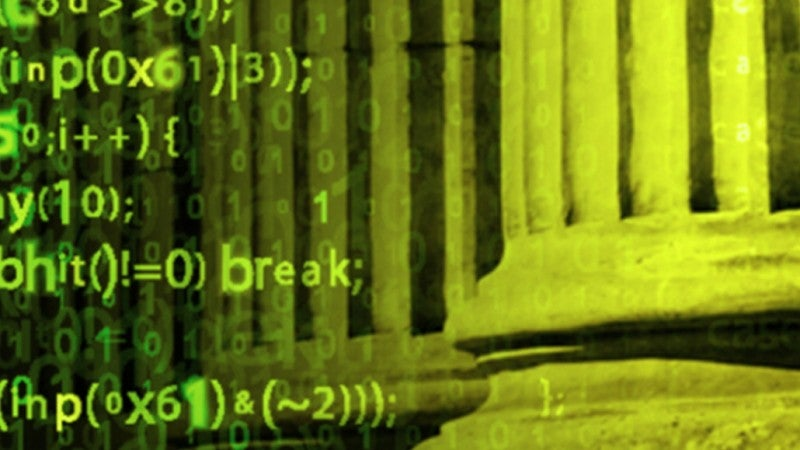This two-part course builds upon the programming skills that you learned in our Introduction to Interactive Programming in Python course. We will augment those skills with both important programming p...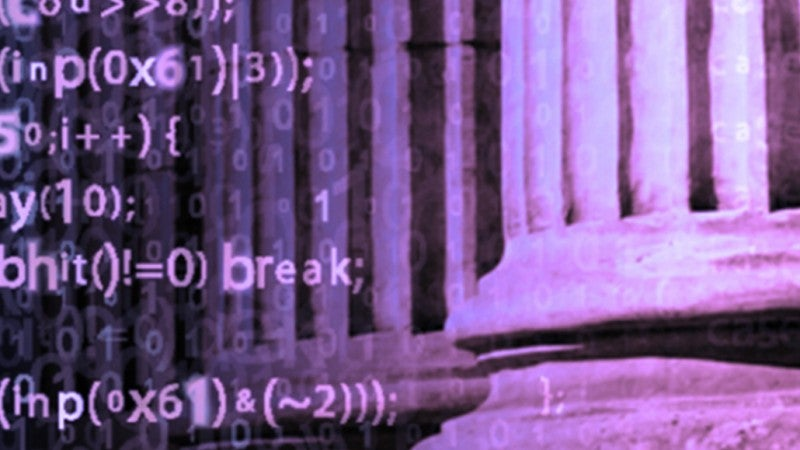This two-part course introduces the basic mathematical and programming principles that underlie much of Computer Science. Understanding these principles is crucial to the process of creating efficient...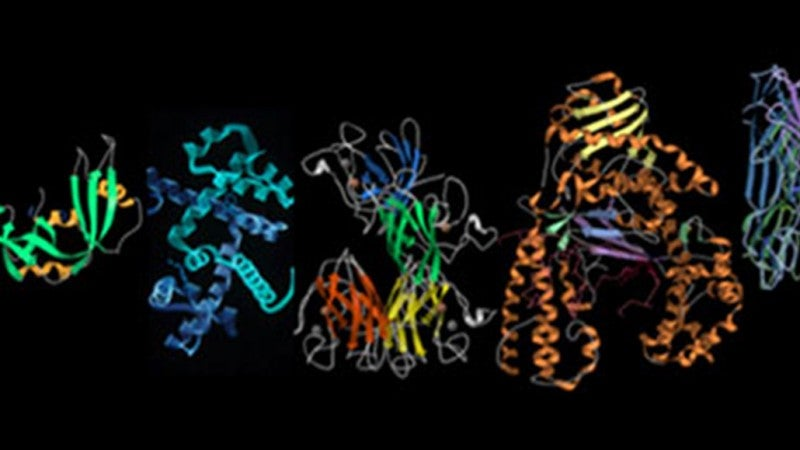Using video lectures, articles, case studies, and molecular models, we will explore how proteins are constructed, how they fold into 3-dimensional shapes, the kinds of bonds that hold these folded str...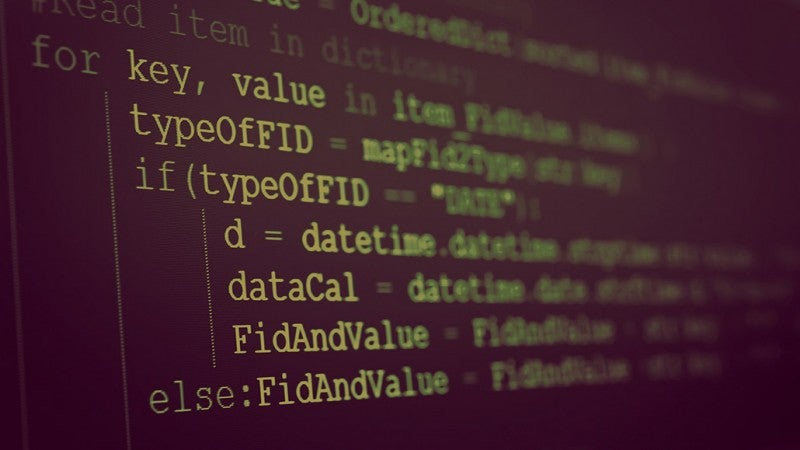Part of the Introduction to Scripting in Python specialization. This course will continue the introduction to Python programming that started with Python Programming Essentials and Python Data Represe...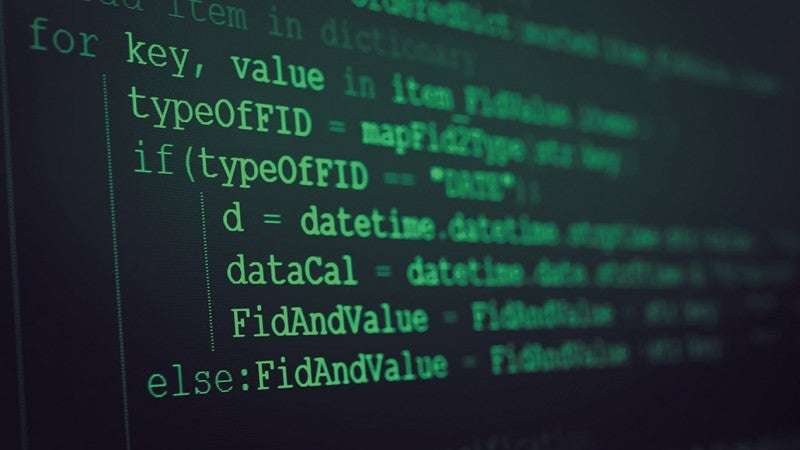Part of the Introduction to Scripting in Python specialization. This course will continue the introduction to Python programming that started with Python Programming Essentials...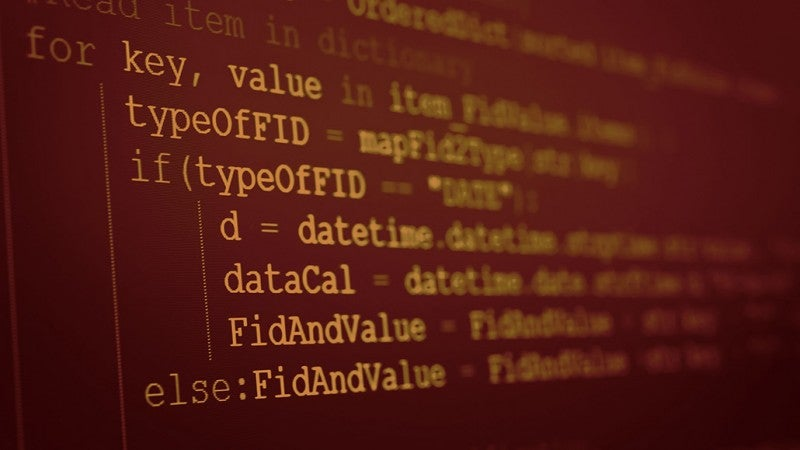Part of the Introduction to Scripting in Python specialization. This if the final course in the specialization which builds upon the knowledge learned in Python Programming Essentials, Python Data Rep...

Body